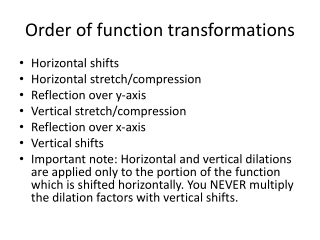DownloadDownload PresentationOrder of function transformations

# Order of function transformations

Télécharger la présentation## Order of function transformations

- - - - - - - - - - - - - - - - - - - - - - - - - - - E N D - - - - - - - - - - - - - - - - - - - - - - - - - - -
##### Presentation Transcript

1. Order of function transformations • Horizontal shifts • Horizontal stretch/compression • Reflection over y-axis • Vertical stretch/compression • Reflection over x-axis • Vertical shifts • Important note: Horizontal and vertical dilations are applied only to the portion of the function which is shifted horizontally. You NEVER multiply the dilation factors with vertical shifts.

2. Order of function transformations • Example: f(x) = x² Shift 2 units left, 4 units up, horizontal stretch by a factor of 6, vertical compression by a factor of ½ and reflect over x-axis. Start with Horizontal shift: (x + 2)² Horizontal stretch: ((1/6)(x + 2))² Vertical compression: ½((1/6)(x + 2))² Reflection over x-axis: - ½((1/6)(x + 2))² Finally, Vertical shift: - ½((1/6)(x + 2))² + 4

3. Order of function transformations • Example: g(x) = x² + 3 Shift 3 units right, reflect across y-axis, stretch vertically by a factor of 2, 2 units up. Start with horizontal shift: (x – 3)² + 3 Reflect across y-axis: (- x – 3)² + 3 Stretch vertically: 2(-x – 3)² + 3 Finally, vertical shift: 2(-x – 3)² +3+2=2(-x -3)² +5

4. Order of function transformations • Now your turn: • Given: x + 2 • Shift 5 units to the right, 3 up, reflect across y-axis, reflect across x-axis, vertical stretch by a factor of 2.